Filip Jerga
March 3, 2021

# Unity Fundamentals — Moving a game object

## Understand transform position and learn simple methods on how to move game objects in Unity.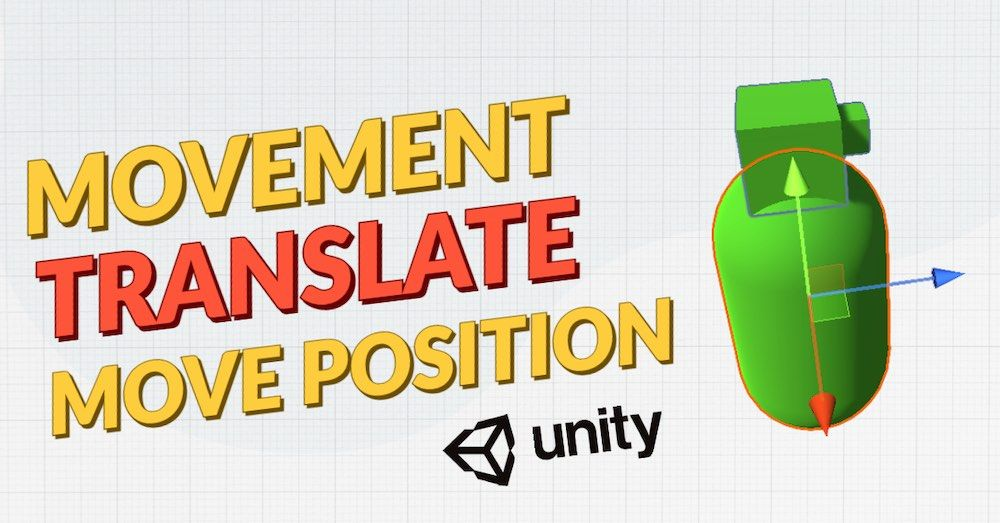## Basics first

Each object in Unity holds Transform. Transform is responsible for position, rotation, and scale manipulation of the object.

Each object placed in the Unity scene has some initial position, rotation, and transform.

The object is residing either in 3D or 2D space, depending on the type of the project. In our case, we will be working on a 3D project.

Let’s take a look at the example of a Capsule object located in 3D space.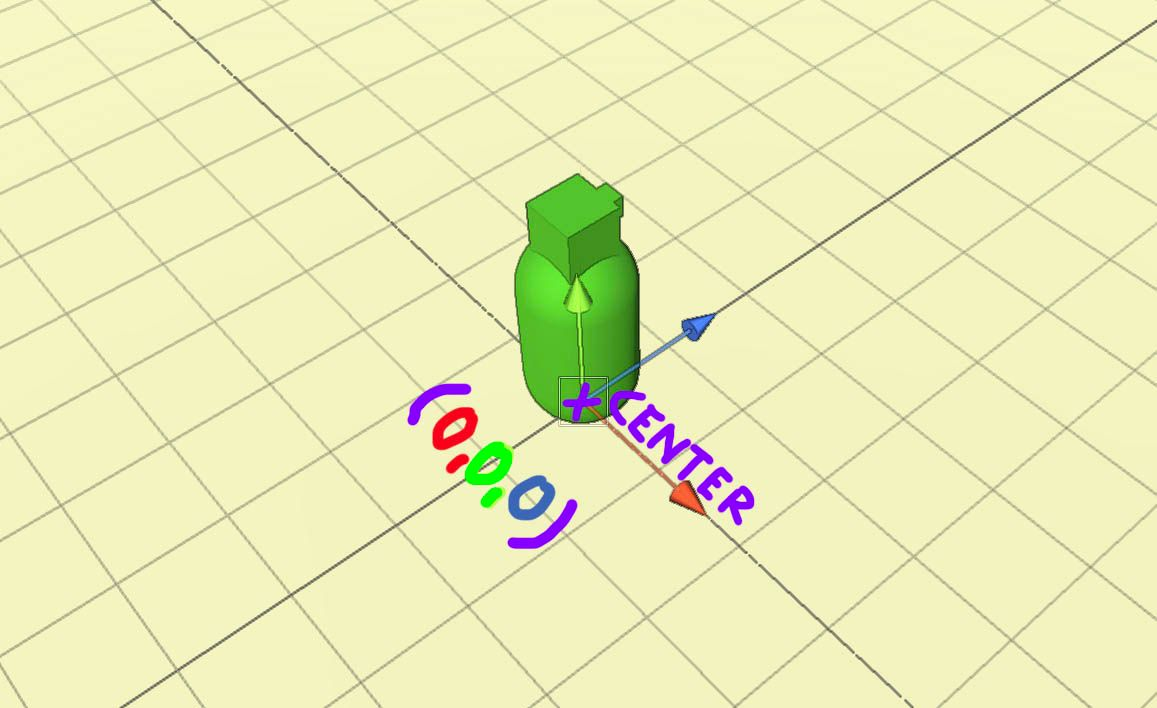The Center of the plane is located at the position (0,0,0) whereas the point in 3d space is determined by 3 values laying on the (X, Y, Z) axis.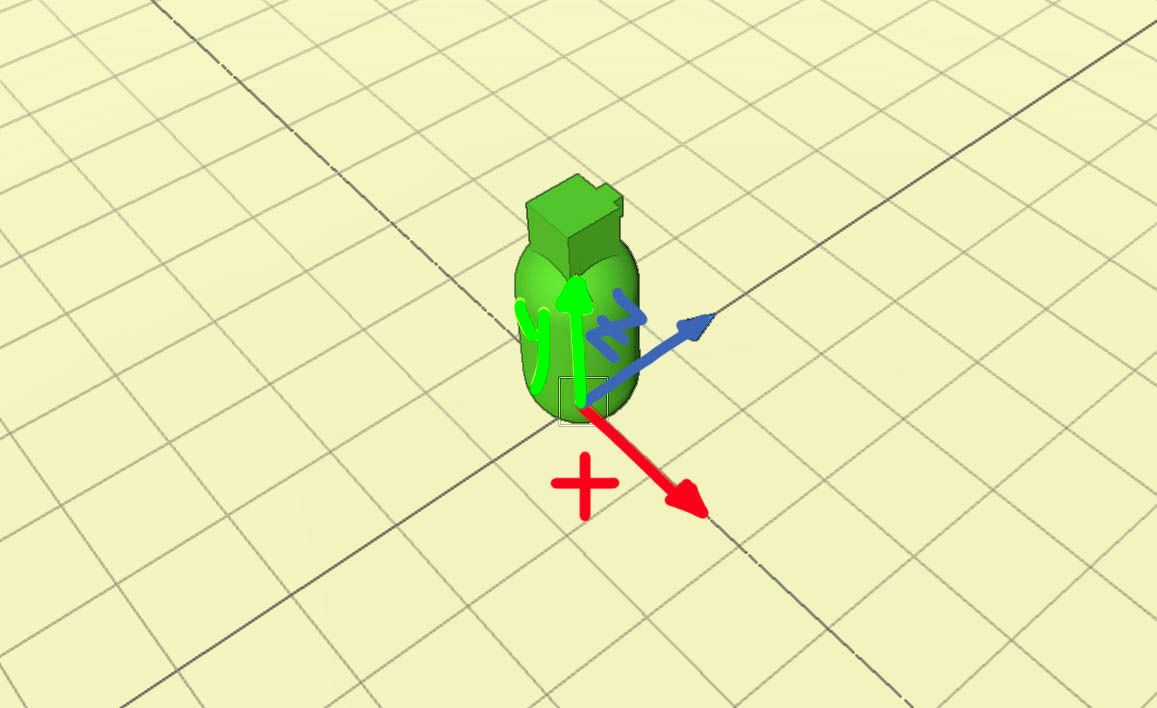Each position on the axis holds a different value. Starting from the center position (0,0,0) going to the right is increasing X value, going upwards Y value is increasing and lastly going forward Z value is increasing. Going in the opposite direction of the mentioned axis causing a decrease of the value.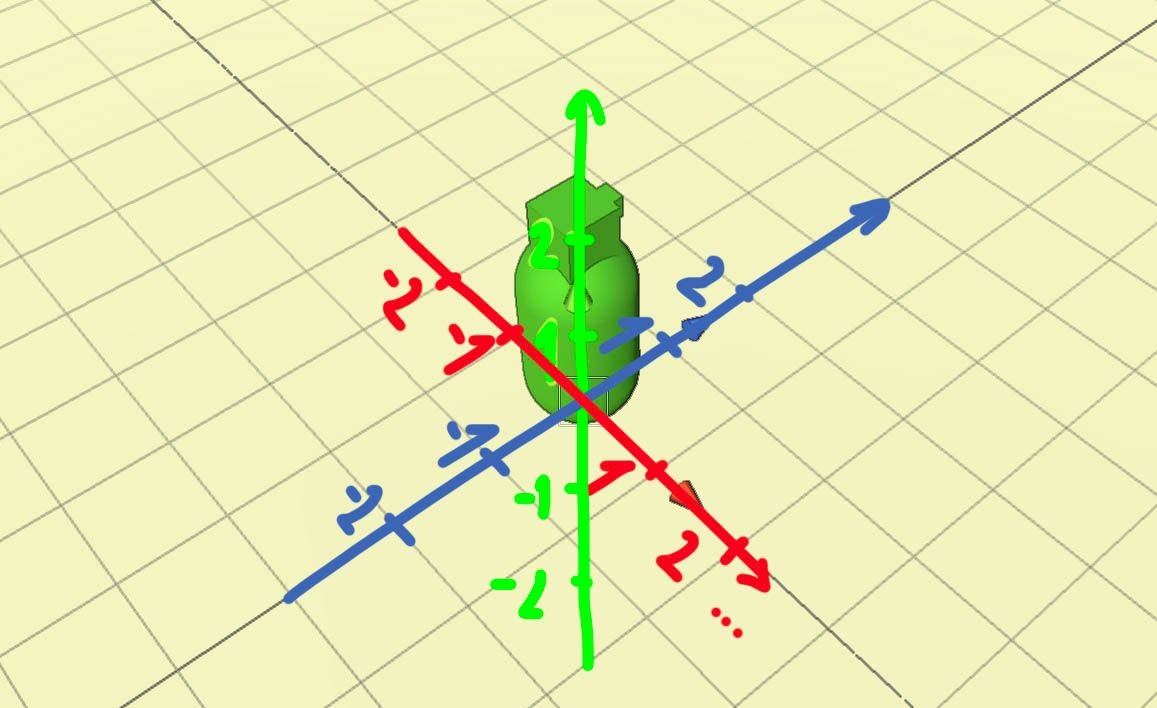In order to find a point in 3D space you need to know all 3 coordinates, (X, Y, Z)

Now let’s see where Capsule would be located at the position (1,1,1)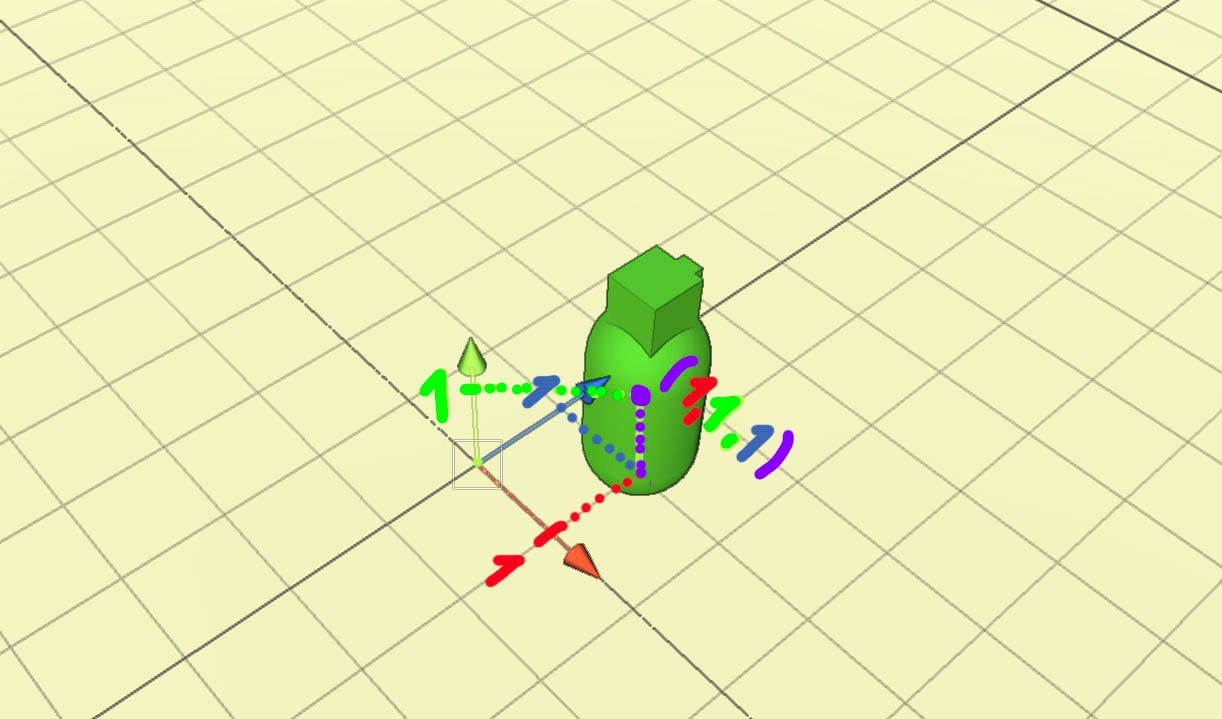Capsule at position (1,1,1)

The Capsule is now located exactly 1 Unity meter on X-axis, 1 meter on the Z-axis, and 1 meter up on Y-axis. Maybe, you are asking the question “Why is the capsule 1 meter on Y?”

Well, the Capsule object has 2 meters, so the center of the capsule is 1 meter above the ground.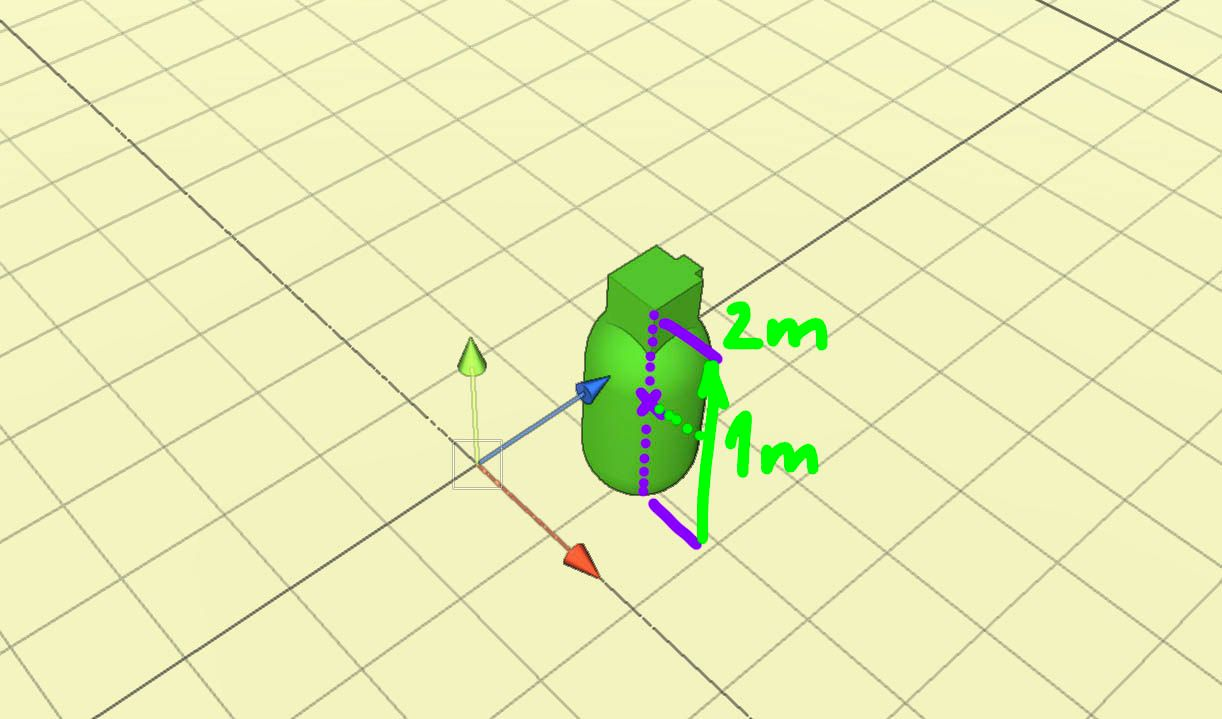Center of the capsule is in the center of the capsule body — 1 meter above the ground

That’s why it needs to be placed 1 meter on the Y-axis. Otherwise, it would be the half-way body in the middle of the plane.

## Change position in the code

You can change the object position in the code by manipulating the transform property of the object.

The position is represented internally by the struct called Vector3. Vector3 holds 3 values -> x,y,z

Let’s move the game object to the position (1,1,1)

`transform.position = new Vector3(1.0f, 1.0f, 1.0f);`

Simple as that!

That’s are the basics you need to know about the position. Remember position is a location in the space represented by the (X, Y, Z) value, each laying on its own axis.

Now we are going to create a functionality to move the Capsule when a player is pressing input keys on the keyboard.

## Movement script

First, create a new script called Movement.cs and add it to the Capsule object.

We will place movement functionality into the Update method. Update is executed during the entire play-time of the game — how often depends on the frame-rate of your game.

The Update method is called once per frame.

If the game is running at 140fps then Update is called 140 times per second. This is making it a great spot for functionality that should happen constantly during the game duration.

Handling player input is usually a functionality that is placed in the Update method.

## Capture a player input

In order to move a game object, we need to capture a player input. If a player is using as the input device a keyboard, we want to capture as he is pressing arrow keys or AWSD keys on the keyboard.

For this purpose, we can write the following code in Update.

``````void Update() {
float horizontalInput = Input.GetAxis("Horizontal");
float verticalInput = Input.GetAxis("Vertical");
Debug.Log("H-> " + horizontalInput);
Debug.Log("V-> " + verticalInput);
}Movement.cs``````

Input values are usually values in the range between -1 and 1. Try it out.

## Horizontal Axis

Horizontal value of 0 means that the NO “movement” key is pressed.

Value 1 means that the player is pressing the “right” arrow or “D” key (player wants to move right)

Value -1 means that the player is pressing the “left” arrow or “A” key (player wants to move left)

## Vertical Axis

Vertical value of 0 means that the NO “movement” key is pressed.

Value 1 means that the player is pressing the “up” arrow or “W” key (player wants to move forward)

Value -1 means that the player is pressing the “down” arrow or “S” key (player wants to move backward)

To get from 0 to 1 or 0 to -1 value while the user is holding down movement keys, will not happen immediately, but with default settings of Unity, it will happen super-fast!

So while holding “right” arrow for one second you can get values in horizontal input, 0, 0.1, 0.3, 0.5, 0.7, 1, 1, 1, 1….reaching 1 value almost imidiately.

These are just illustrational values, you can get completely different ones.

Since we can capture the player input we are almost ready to move.

Let’s extend the current implementation.

``````void Update() {
float horizontalInput = Input.GetAxis("Horizontal");
float verticalInput = Input.GetAxis("Vertical");
Vector3 movement = new Vector3(horizontalInput, 0, verticalInput);
transform.Translate(movement);
}Movement.cs``````

We are moving!

You will notice one thing. We are moving too fast.

First, let’s explain the code above.

We are storing the vertical and horizontal values in the Vector3 structure, in order to apply them to change the game object position.

As I mentioned before, every game object has a position represented by (X, Y, Z) value.

We need to apply this change of position in form of Vector3 and then provide it to the Translate method. The Translate method will move a game object in direction and distance of translation.

Imagine these 3 cases:The object will move right when a player is holding the “right” arrow or “D” because Vector3 is holding value (1,0,0), which expresses a change in direction on X-axis.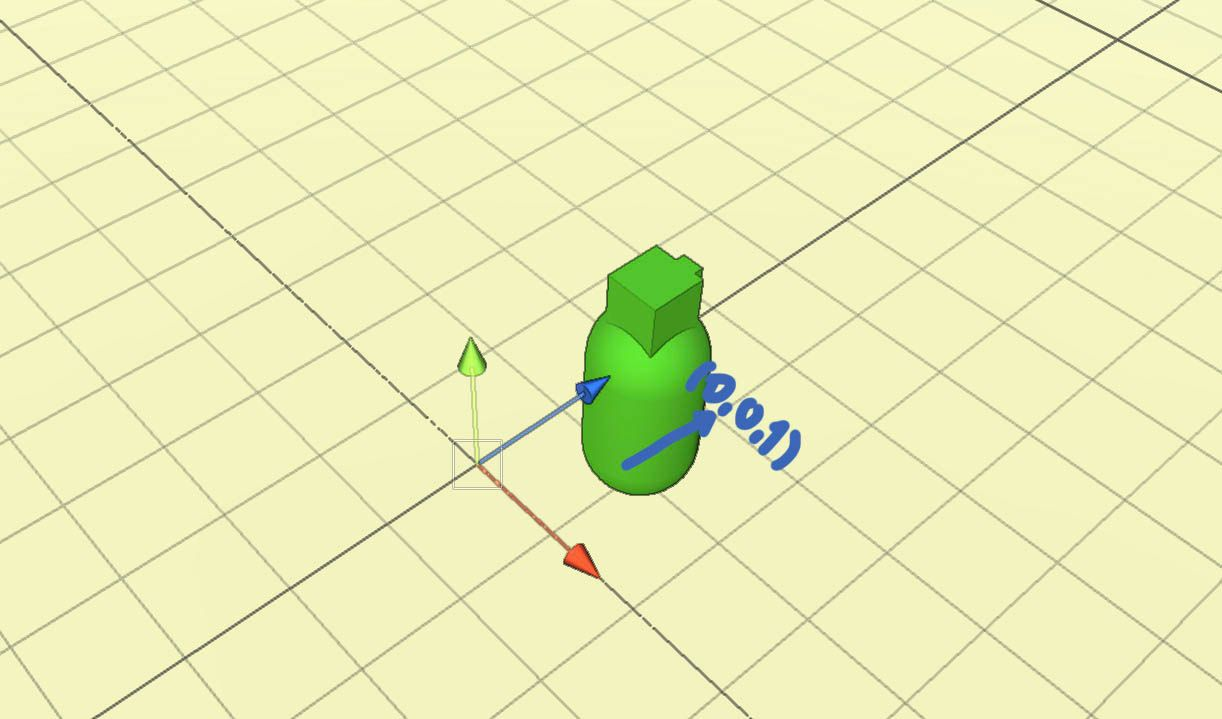The object will move forward when a player is holding the “up” arrow or “W” because Vector3 is holding a value (0,0,1), which expresses a change in direction on Z-axis.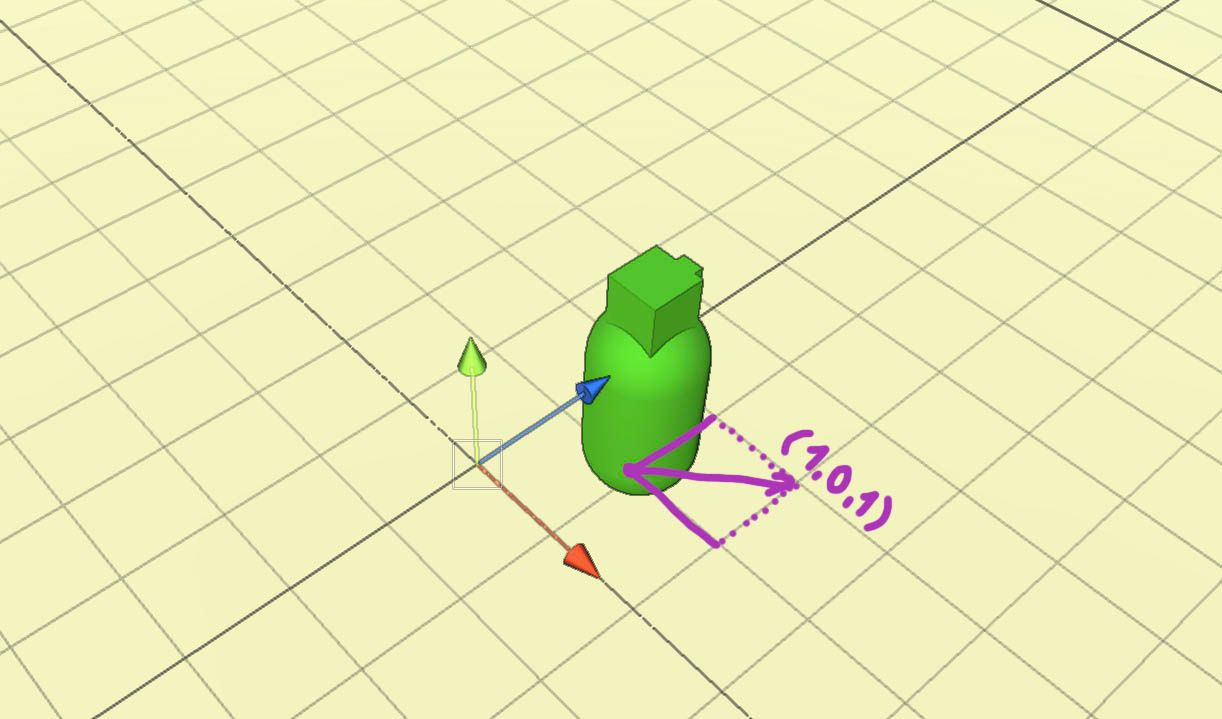The object will move diagonally when a player is holding the “up” arrow or “W” at the same time as the “right” arrow or “D” key, because Vector3 is holding value (1,0,1), which expresses a change in direction on X and Z axis.

These changes are applied to the game object’s current position. You can think of the game object as the center of the plane.

## Why is the object moving fast?

The game object is moving fast because Update is called many times per one second. Imagine stable frame-rate(FPS) to be 50. 50 FPS means that your game is updated 50 times per second therefore Update is called 50 times per second.

This also means that holding input keys for the length of 1 second would result in 50 executions of

``````Vector3 movement = new Vector3(horizontalInput, 0, verticalInput);
transform.Translate(movement);Movement.cs``````

so if Vector3 would be (1,0,0) then I would move each second 50 meters to the right because Update is called 50 times per second.

Our functionality is frame-dependent. In order to move only 1 meter per second, we need to decrease the value of the movement.

## Time delta

First, update translate like this

`transform.Translate(movement * Time.deltaTime);`

If you will play the game now you will notice that movement is fixed and you are moving nice and slowly.

Time delta is the completion time of the last frame in seconds.

Again, imagine a stable frame-rate of 50 frames per 1 second. Completion time of each frame would be 0.02 second because 50 * 0.02 = 1 second

If you multiple the movement by 0.02 you will lower its value, if this happens 50 times per second then Vector3(0,0,1) will apply movement of 1 meter per second.

Now the functionality of the movement is time-dependent. You will use Time.deltaTime a lot.

## Final improvements

If the movement is too slow then multiple the movement with some higher value. Let’s say you want to move the Capsule to move 6 meters per second.

Vector3 movement = new Vector3(horizontalInput, 0, verticalInput);
transform.Translate(movement * 6.0f * Time.deltaTime);

That’s it.

Now the final code.

``````void Update() {
float horizontalInput = Input.GetAxis("Horizontal");
float verticalInput = Input.GetAxis("Vertical");
Vector3 movement = new Vector3(horizontalInput, 0, verticalInput);
movement.Normalize();
transform.Translate(movement * 6.0f * Time.deltaTime);
}Movement.cs``````

You can notice I called Normalize method on movement. In the case of diagonal movement, you will move faster. Normalize will “fix” it and movement will be the same in every direction.

I am explaining Normalize in much more detail in my other Youtube video: https://www.youtube.com/watch?v=oCU8Ew1XTbs&t=3s

## Recap

Now you should have a basic understanding of how to move a game object in Unity. Everything is just about the change of the position over some time-interval.

Make sure to check also other resources: